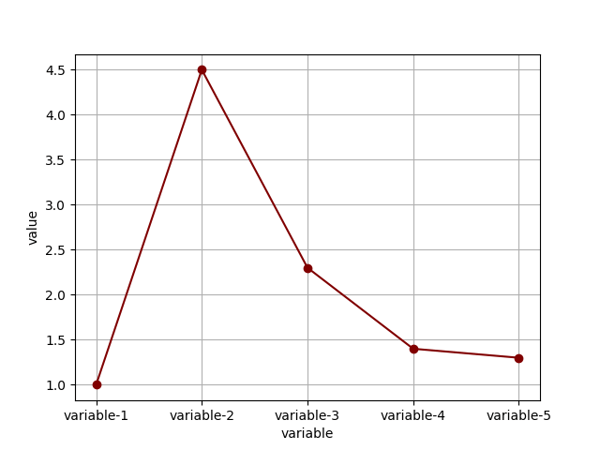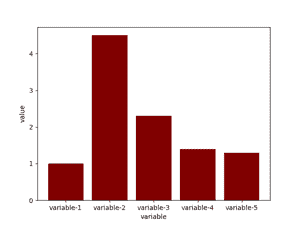Related Tags

json
python
data visualization
communitycreator

# How to plot graphs using .json files in Python

JSON files can be leveraged to collect data to be processed in Python. This is because the Python dictionaries have the same format as JSON and can easily be converted from one to another.

### Syntax

The format of a .json file is as follows:

{
"variable-1": 1.0,
"variable-2": 4.5,
"variable-3": 2.3,
"variable-4": 1.4,
"variable-5": 1.3
}

Now, we can store this .json file in a Python dictionary and plot graphs of multiple kinds using the matplotlib library. JSON files can be stored in a python dictionary using the json.load() function.

Below is an example of a Python script creating a line graph and a bar graph using data from a .json file:

main.py
file.json
import matplotlib.pyplot as plt
import json

xAxis = [key for key, value in dictionary.items()]
yAxis = [value for key, value in dictionary.items()]
plt.grid(True)

## LINE GRAPH ##
plt.plot(xAxis,yAxis, color='maroon', marker='o')
plt.xlabel('variable')
plt.ylabel('value')

## BAR GRAPH ##
fig = plt.figure()
plt.bar(xAxis,yAxis, color='maroon')
plt.xlabel('variable')
plt.ylabel('value')

plt.show()

The python script above outputs the following graphs:Line GraphBar Graph

RELATED TAGS

json
python
data visualization
communitycreator

CONTRIBUTOR